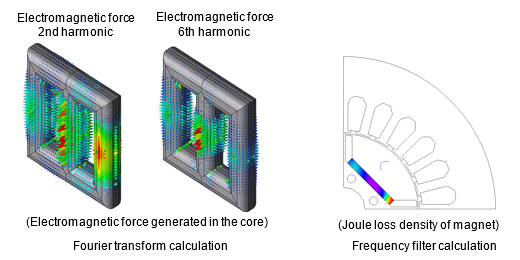## [JFT023] Frequency Component Analysis of Distributions Obtained by Transient Response Analysis

Remember me

*It is different from the service for JMAG WEB MEMBER (free member). Please be careful.
About authentication ID for JMAG website

### Overview

When understanding complicated phenomena, it is sometimes useful to check analysis results by focusing on specific frequency components. JMAG can convert the distribution obtained by transient analysis to the frequency domain.
This document shows how to display output results ij vector form using the Fourier transform calculation function.Fig.a Fourier transform calculation and frequency filter calculation

Table.a Distribution Analysis Function

Function name Characteristic
Fourier transform calculation ・Converts time domain results to frequency domain.
・Used when evaluating the distribution at a specific frequency.
Frequency filter calculation ・Extract only specified frequency components from the time domain and convert them back to the time domain.
・ Used when evaluating of the distribution at each time step for a given frequency and position on a part.

#### Keywords

Fourier transform calculation, Fourier transform (distribution), frequency filter calculation, frequency component, distribution display, counter plot, vector plot